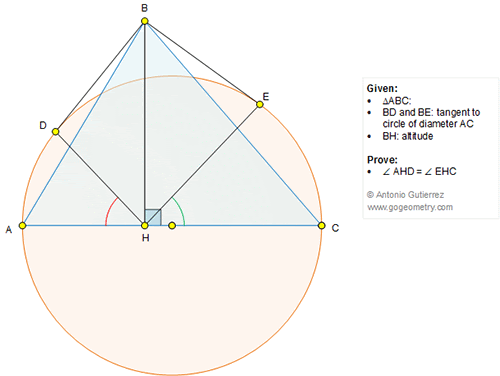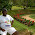## Sunday, October 30, 2016

### Geometry Problem 1281 Triangle, Circle, Diameter, Tangent, Altitude, Congruent Angles

Geometry Problem. Post your solution in the comment box below.
Level: Mathematics Education, High School, Honors Geometry, College.

Click the figure below to view more details of problem 1281.1.https://goo.gl/photos/cZVha9LddjDaWn519

Connect OB, OD ,OE where O is the midpoint of AC
We have OD⊥BD and OE⊥BE and OB is the angle bisector or angle BDE
So O, H, D, B, E are cocyclic
In circle OHDBE ∠AHD= ∠DBO
∠CHE=∠OHE= ∠OBE
But ∠DBO=∠OBE => ∠AHD=∠CHE

2.Let AD, HB meet at P. Let HPB extended meet AD extended at X.

Now P is the orthocenter of triangle AXC, hence AP is perpendicular to XC.
But AE is perpendicular to XC. It follows that A,P,E are collinear and XEC
are collinear.

P being the orthocenter of triangle AXC, easily P is the incentre of
triangle HDE.

So < AHD = < CHE

Sumith Peiris
Moratuwa
Sri Lanka

3.Problem 1281

Suppose that the DC intersect BH in point P and AD the BH to Q.Then < DCA=<QDB ( cord and tangent) and <DQB=<DCA (perpendicular),so <DQB=<QDB or BD=BQ=BE. But <PDB=90-<BDQ=<DPB,then BD=BP=BE=BQ. So PE is perpendicular to BE.
Then at triangle PAC the point P is orthocenter, and A,P and E
Are collinear. The ADPH ,HPEC are concyclic. So <DHA=<DPA=<EPC=<EHC.
4 HIGH SCHOOL OF KORYDALLOS PIRAEUS-GREECE

4.m(BOD) = mBOE) = m(BOH) = 90 => B,D,H,O & E are concylic
Since BD = BE => m(BHD) = m(BHE) = @ (say)
Therefore m(AHD) = m(CHE) = 90-@